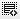## Assume you are a banker advising a client with a Debt/Equity ratio of 5x. The client's interest coverage is 1.3x and they are expecting a 10% decrease0
I need a step by step calculation for this question.0

This is a quiz question so I cannot give you the answer in this public forum. However I will try to point you in the right direction.

at present operating income is 1.3 times of present interst expense (10*1.3 = 13 crore), now in coming year it would decrease by 10% as per question hence it would become 11.7 crore(13*.90 = 11.7).
now how much more interest company can bear can be worked out
which is like this ==>> 11.7 - 10 = 1.7crore.

now to pay up to 1.7 crore we can take this much loan@10%
1.7/10 * 100 = 17 crore.

Click on thisicon to add code snippet.